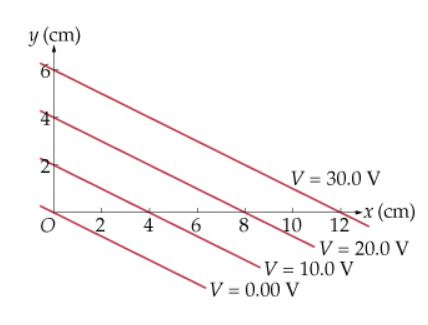# Problem: A given system has the equipotential surfaces shown in the figure (Figure 1).a) What is the magnitude of the electric field? (V/m)b) What is the direction of the electric field? ( ∘ from the +x axis )

###### FREE Expert Solution

Electric field components:

$\overline{)\begin{array}{rcl}{\mathbf{E}}_{\mathbf{x}}& {\mathbf{=}}& \mathbf{-}\frac{\mathbf{∆}\mathbf{V}}{\mathbf{∆}\mathbf{x}}\\ {\mathbf{E}}_{\mathbf{y}}& {\mathbf{=}}& \mathbf{-}\frac{\mathbf{∆}\mathbf{V}}{\mathbf{∆}\mathbf{y}}\end{array}}$

The magnitude of the electric field:

$\overline{){\mathbf{E}}{\mathbf{=}}\sqrt{{{\mathbf{E}}_{\mathbf{x}}}^{\mathbf{2}}\mathbf{+}{{\mathbf{E}}_{\mathbf{y}}}^{\mathbf{2}}}}$

Direction:

$\overline{){\mathbf{\theta }}{\mathbf{=}}{\mathbf{t}}{\mathbf{a}}{{\mathbf{n}}}^{\mathbf{-}\mathbf{1}}{\mathbf{\left(}}\frac{{\mathbf{E}}_{\mathbf{y}}}{{\mathbf{E}}_{\mathbf{x}}}{\mathbf{\right)}}}$

93% (283 ratings)###### Problem Details

A given system has the equipotential surfaces shown in the figure (Figure 1).a) What is the magnitude of the electric field? (V/m)

b) What is the direction of the electric field? ( ∘ from the +x axis )